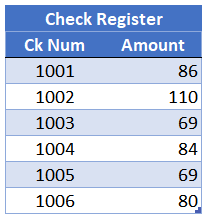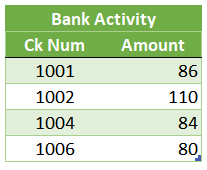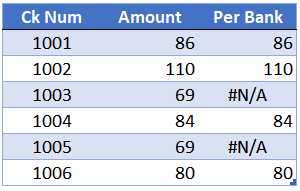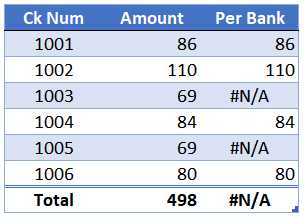# VLOOKUP Hack #3: Exact MatchIn this post, the 3rd in the VLOOKUP Hacks series, we’ll dig even deeper into the 4th argument. We’ll see how we can leverage it to help with our reconciliations and other list comparisons.

Let’s pretend for a moment that we need to compare two lists. We’d like to find out which items in one list appear in the other list. This type of thing is often referred to as a list comparison. Accountants often refer to this task as a reconciliation. So, let’s use VLOOKUP to complete one of the most common reconciliations of all, a bank rec.

I’ve prepared a video demonstration as well as the detailed steps below for reference.

## Detailed Steps

In a bank rec, one list is the check register and the other list is the bank activity. Here is a way-simplified check register:And here is the activity per the bank that we downloaded from the bank website:Now, we have a simple question. Which checks in the check register have cleared the bank? That is, which checks on the check register are also found in the bank activity table.

When a check is found in the bank activity table, we want to return the amount. This sounds like a job for VLOOKUP.

So, we write the following formula

`=VLOOKUP([Ck Num],Table1,2)`

We get these results:Specifically, we get an amount for every check, even those that haven’t cleared the bank! For example, checks 1003 and 1005 are not in the bank table, but, the formula retrieves a value for them. So, what are we supposed to do? Do the bank rec manually? No! This brings us to our next hack.

## Hack

In the formula above, the 4th argument is omitted, so, it is performing a range lookup. We discussed this in detail in the previous post. When VLOOKUP performs a range lookup, it is not looking for an exact matching value. Instead, it is looking for a value that falls between a range of values. For example, 1003 returns 110 from the bank activity table because 1003 is >=1002 and <1004. Upon reflection, this is exactly what we would expect after understanding range lookups from the previous post. Now, here’s the hack that will help.

Hack: when the 4th argument is FALSE, VLOOKUP returns #N/A when no matching value is found!

So, let’s modify the formula above by using FALSE (or 0) as the 4th argument:

`=VLOOKUP([Ck Num],Table1,2,FALSE)`

Yes … it worked! The update is shown below.Now, we can easily see which checks have cleared the bank (those where the amount per bank is returned), and which are still outstanding (#N/A).

So, we are good, right? Well, not so fast. You see, the #N/A error will trickle down to mess up any formulas that include the range. For example, perhaps we want to compute a total, as shown below.So, is there a way to clean up the #N/A errors, so that the reconciliation will look cleaner and so that the total formula won’t be broken? Yes…we can get a little help from the IFERROR function.

In summary, IFERROR will return its first argument, unless it is an error. If so, it will return the second argument instead.

So, we can replace the #N/A with something else. For example, we could replace it with 0 like this:

`=IFERROR(VLOOKUP([Ck Num],Table1,2,FALSE),0)`

Or, we could replace it with a text string by enclosing the text string in quotes, like this:

`=IFERROR(VLOOKUP([Ck Num],Table1,2,FALSE),"Outstanding")`

The results are below.Now it is very clear which checks are still outstanding, and the total works!

Wow, that tiny little 4th argument has a big impact on how the VLOOKUP function behaves, and, opens up many interesting way we can apply it to our work.

Get a quick email notice when a new Excel article is available

• This field is for validation purposes and should be left unchanged.1.Adina

Hello Mister Lenning,
First, thanks for all the tricks…is very interesting and helpful.
I wish to k now, please, in this Vlookup formula , VLOOKUP([Ck Num],Table1,2), how you put the [@Ck Num] instead of D7 like in your exemple?
is very useful but i don’t know how to do it.
Thank you
1.Jeff Lenning Post author
2.Denise